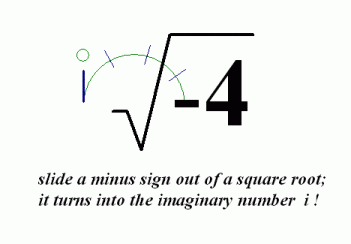﻿ The Square Root of a Negative Number

THE SQUARE ROOT OF A NEGATIVE NUMBER

• PRACTICE (online exercises and printable worksheets)

Square roots of negative numbers were introduced in a prior lesson:
Arithmetic with Complex Numbers in the Algebra II materials.
For your convenience, that material is repeated (and extended) here.

Firstly, recall some information from beginning algebra:

The Square Root of a Nonnegative Real Number

For a nonnegative real number $\,k\,$, $$\overbrace{\ \ \ \sqrt{k}\ \ \ }^{\text{the square root of \,k\,}} := \ \ \text{the unique nonnegative real number which, when squared, equals \,k\,}$$

(Note: when the distinction becomes important in higher-level mathematics, then $\,\sqrt{k}\,$ is called the principal square root of $\,k\,$.)

Thus, the number that gets to be called the square root of $\,k\,$ satisfies two properties:

• it is nonnegative (not negative; i.e., greater than or equal to zero)
• when squared, it gives $\,k\,$
For example, $\,\sqrt{9} = 3\,$ since the number $\,3\,$ satisfies these two properties:
• $\,3\,$ is nonnegative
• $\,3\,$, when squared, gives $\,9\,$:   $\,3^2 = 9\,$

So, what is (say) $\,\sqrt{-4}\$?
There does not exist a nonnegative real number which, when squared, equals $\,-4\$.
Why not?
Every real number, when squared, is nonnegative:   for all real numbers $\,x\,$,   $\,x^2 \ge 0\,$.
Complex numbers to the rescue!

The Square Root of a Negative Number

Complex numbers allow us to compute the square root of negative numbers, like $\,\sqrt{-4}\$.
Remember the key fact:   $\,i:=\sqrt{-1}\,$,   so that   $\,i^2=-1\,$

THE SQUARE ROOT OF A NEGATIVE NUMBER
Let $\,p\,$ be a positive real number, so that $\,-p\,$ is a negative real number. Then: $$\overbrace{\ \ \ \sqrt{-p}\ \ \ }^{\text{the square root of negative \,p\,}} \ :=\ i\,\sqrt{\vphantom{h}p}$$
(Note: when the distinction becomes important in higher-level mathematics, then $\,\sqrt{-p}\,$ is called the principal square root of $\,-p\,$.)

Observe that $\,i\sqrt{\vphantom{h}p}\,$, when squared, does indeed give $\,-p\$: $$(i\sqrt{\vphantom{h}p})^2 \ =\ i^2(\sqrt{\vphantom{h}p})^2 \ =\ (-1)(p) \ =\ -p$$

Some of my students like to think of it this way:
You can slide a minus sign out of a square root, and in the process, it turns into the imaginary number $\,i\,$!Here are some examples:

• $\sqrt{-4} = i\sqrt{4} = i2 = 2i\,$

It is conventional to write $\,2i\,$, not $\,i2\,$.
When there's no possible misinterpretation (see below), write a real number multiplier before the $\,i\,$.

• $\sqrt{-5} = i\sqrt{5}$

In this situation, it's actually better to leave the $\,\sqrt{5}\,$ after the $\,i\,$.
Why?   If you pull the $\,\sqrt{5}\,$ to the front, it can lead to a misinterpretation, as follows:
The numbers $\,\sqrt{5}i\,$ and $\,\sqrt{5i}\,$ are different numbers—look carefully!
In $\ \sqrt{5}i\$, the $\,i\,$ is outside the square root.
In $\ \sqrt{5i}\$, the $\,i\,$ is inside the square root.
Unless you look carefully, however, you might mistake one of these for the other.
By writing $\,i\sqrt{5}\,$, you eliminate any possible confusion.

• TWO DIFFERENT QUESTIONS; TWO DIFFERENT ANSWERS

Recall these two different questions, with two different answers:

• What is $\,\sqrt{4}\,$?
Answer:   By definition, $\,\sqrt{4} = 2\,$.
The number $\ \sqrt{4}\$ is the nonnegative number which, when squared, gives $\,4\,$.
• What are the solutions to the equation $\,x^2 = 4\,$?
Answer: $\,x= \pm\ 2\,$
There are two different real numbers which, when squared, give $\,4\,$.

Similarly, there are two different questions involving complex numbers, with two different answers:

• What is $\,\sqrt{-4}\,$?
Answer:   $\sqrt{-4} = 2i\,$
• What are the (complex) solutions to the equation $\,x^2 = -4\,$?
Answer: $\,x=\pm \sqrt{-4} = \pm\ 2i\,$
There are two different complex numbers which, when squared, give $\,-4\,$.
Verifying the second solution: $\,(-2i)(-2i) = 4i^2 = 4(-1) = -4$

Square Roots of Products

The following statement is true:   for all nonnegative real numbers $\,a\,$ and $\,b\,$, $\,\sqrt{ab} = \sqrt{a}\sqrt{b}\$.
For nonnegative numbers, the square root of a product is the product of the square roots.

Does this property work for negative numbers, too?
The answer is NO, as shown next.

Certainly, anything called ‘the square root of $\,-4\,$’ must have the property that, when squared, it equals $\,-4\$.
Unfortunately, the following incorrect reasoning gives the square as $\,4\,$, not $\,-4\,$: $$\text{? ? ? ? }\ \ \ \ (\sqrt{-4})^2 \ \ =\ \ \sqrt{-4}\sqrt{-4} \overbrace{\ \ =\ \ }^{\text{this is the mistake}}\ \ \sqrt{(-4)(-4)}\ \ =\ \ \sqrt{16}\ \ =\ \ 4\ \ \ \ \text{? ? ? ? }$$ Here is the correct approach: $$(\sqrt{-4})^2 \ \ =\ \ \sqrt{-4}\sqrt{-4}\ \ =\ \ i\sqrt{4}\ i\sqrt{4} \ \ =\ \ i^2(\sqrt{4})^2 \ \ =\ \ (-1)(4) \ \ =\ \ -4$$

Similarly, $\,\sqrt{-5}\sqrt{-3}\,$ is NOT equal to $\,\sqrt{(-5)(-3)}\,$.
Instead, here is the correct simplication: $$\sqrt{-5}\sqrt{-3} \ \ =\ \ i\sqrt{5}\ i\sqrt{3} \ \ =\ \ i^2\sqrt{5}\sqrt{3} \ \ =\ \ (-1)\sqrt{(5)(3)} \ \ =\ \ -\sqrt{15}$$ Be careful about this!

Master the ideas from this section